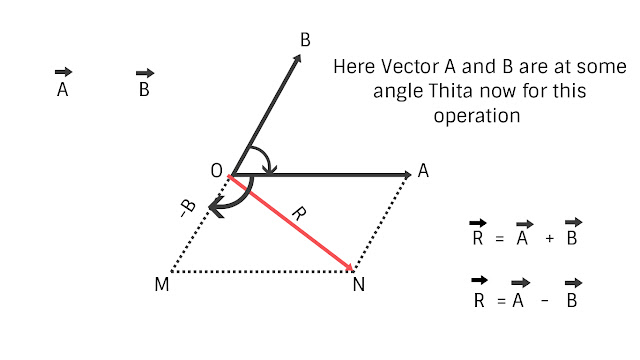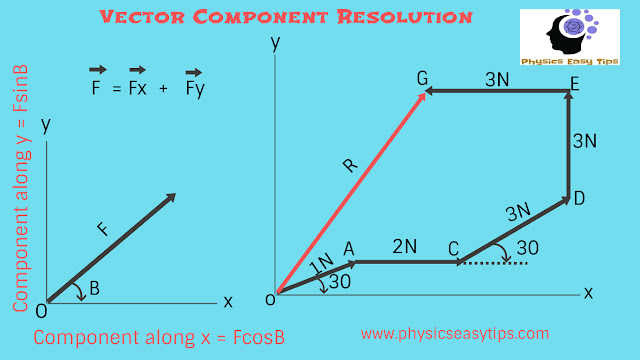Vector addition subtraction and componentSubtraction of vector: i will suggest you first go and study concept of addition of vectors above mentioned link because to understand subtraction you must understand addition of vector because subtraction of vector is actually addition of vector understand concept vector follow only addition operation no subtraction operation nothing is subtraction in vector has no meaning then why we study subtraction method the reason behind this is only due to direction magnitude is fixed in any vector but direction can be change and may be in any direction take an example to understand concept suppose i told John has moved 5m here 5m is magnitude here it is not specified in which direction John has moved it can be left , right, up, down or some angle in any of the direction from the original position or origin 5m is scalar until direction is not specified now suppose i told John has moved 5m in right now it is vector because direction is specified here if i take this direction positive then opposite of this means left will be negative now for vector representation. →                                    → A right is positive then  -A vector will be in left direction. suppose there are two vector A and vector B given below direction.               →                                            →                              A                                            -A→→→→→→ (+ve direction)   ←←←←←← (-ve direction)            →                                               →          B                                                -B→→→→→→ (+ve direction)   ←←←←←← (-ve direction)now do the operation for two vector  A and B let R is result →      →     →R    = A   +  B   ( operation is addition )→      →      →      →       →R    = A    –    B  = A   +  (-B)   (operation is addition B vector -ve)hence it is clear that only operation performed in both the cases is addition whereas we say second case subtraction but it is actually addition so vector can only be added not subtracted.hence it is called negative of vector magnitude remains same but direction changes opposite of original direction. Two vector addition at some angle.here from triangle ∠BOA = ? then in triangle ∠AOM = 180-? OM is -B vector opposite of B vector so for A-B vector operation extended OM and using parallelogram method tail- tail addition for resultant and here resultant for vector A- B will be R²  = A² +B²+2ABcos(180-?) now we know that cos(180-?) = -cos? here important to understand that -B is always taken positive because in parallelogram A and B are side of parallelogram it can never be negative always put positive value and take angle between -B vector and A vector hence final resultant is  R²  = A² +B²-2ABcos?. now we will do a small numerical question . Q A car run at 10 m/s east then take a sharp turn towards north and continue with 10 m/s find the change in velocity of car. Ans (a )10√2 SW  (b) 0 SE  (c) 10√2 NE  (d) 10√2 NW here four option is given every option have same magnitude but direction is different why direction because velocity is a vector quantity. here we have to find the change in velocity we know that change in velocity. ∆v  = final velocity – initial velocity = 10 -10 = 0 we can’t do like this because velocity is a vector quantity we have to do here vector operation see figure below .                                         N                                         ↑                                                                          ↑ 10 m/s final                                         ↑                     W←←←←↓→→→→→E                                         ↓      10 m/s  initial                                         ↓                                          ↓                                         ↓                                         S    →        →    →       →        →  ∆v     = V₂ – V₁   = V₂  + (-V₁ )  ( addition operation) use formula for magnitude calculation      ∆v²  =   V₂²  + V₁²   -2V₂V₁cos90  here angle between V₂ and V₁     ∆v²  = 10² +10² -0 so ∆v = 10√2  now for direction V₁ vector is east  V₂ vector is north hence -V₁ vector direction will be west hence change in velocity vector ∆v will be NW so answer is 10√2 NW m/s not NE proper follow vector direction. Resolution or component of vector :just we have study that combining two vector makes a single resultant vector similarly whenever a vector acting at an angle can be resolve in two component in any plan along x axis and along y axis concept even a single vector acting along x axis has two component where as its y component is zero our motive is to break any vector along two component for vector addition in plane by this method we can add many vector easily by adding all component along x axis and y axis separately and for final resultant add total component of x axis and total component of y axis by vector method and this will give final resultant or result whenever a vector acting along x axis and along y axis no need to break component because it is already component along x axis and y axis only we need to break those vector component which are not along x axis and y axis but acting at some angle. see picture below for vector component.As stated above any angled vector can be resolve in two component and then add easily many vector see in picture five force vector we will add and find the resultant here five vector are OA, AC,CD,DE,EG now follow the vector component rule to add all vector first we will take all five vector x component then y component hence Fx denote component along x axis see how we calculate take magnitude and angle of cos → Fx  = 1Cos30i^ +2i^+ 3cos30i^ + 0  – 3i^ here 0 its component along x axis is zero -3 along negative direction i^ is unit vector along x axis now simplify → Fx  = √3/2i^ +3√3/2i^ -i^ = 4√3/2i^ -i^ = 2.464i^ now similarly take component for all vector along y axis → Fy  = 1sin30j^ +0 +3sin30j^ + 3j^ +0  here j^ is unit vector along y axis now simplify → Fy   = 1/2j^ +3/2j^ +3j^ = 5j^ hence total resultant R →     →    → R   = Fx  +Fy  = 2.464i^ + 5j^ hence for magnitude ∣R∣  = √(2.464)² +25  = 5.57 N since this is force hence it has direction so for angle apply tan? = 5/2.464. now through this method any number of vector can be added easily  we will see more question of vector in every topic thanks for reading Vector addition subtraction and component    dated 10th Jun 2018